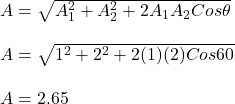## “Two waves of the same frequency have amplitudes 1.00 and 2.00. They interfere at a point where their phase difference is 60.0°. What is the

Question

“Two waves of the same frequency have amplitudes 1.00 and 2.00. They interfere at a point where their phase difference is 60.0°. What is the resultant amplitude?”

in progress 0
5 months 2021-08-09T20:07:26+00:00 1 Answers 54 views 0

## Answers ( )

The resultant amplitude of the two waves is 2.65.

Explanation:

Given;

amplitude of the first wave, A₁ = 1

amplitude of the second wave, A₂ = 2

phase difference of the two amplitudes, θ = 60.0°.

The resultant amplitude of two waves after interference is given by;Therefore, the resultant amplitude of the two waves is 2.65.